10

# 神經網路

• 二元一次線性方程式ax + by + c，在神經網路x即是神經元，a和b是權重，c是偏權值(移動量10如下圖)，輸出分為兩類等於0或小於0，因此公式為(x * w) + c若小於0則輸出0，若大於等於0輸出1。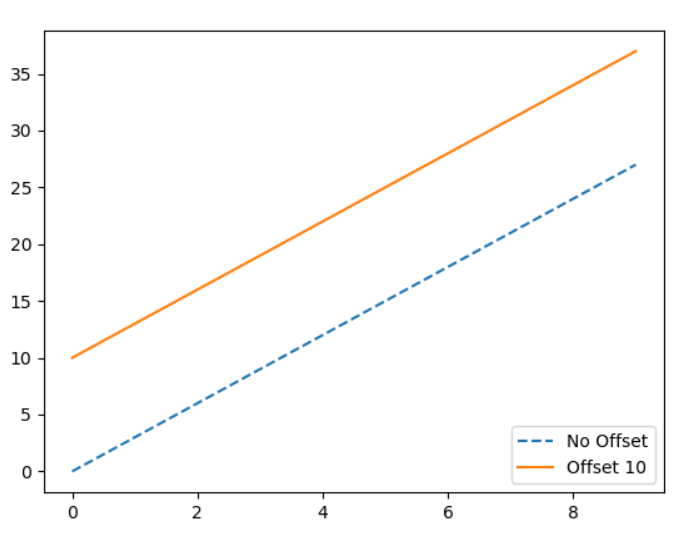• 一般神經網路的輸入可能為多組向量(批量處理)，然而權重相對也是多組向量，因此在神經網路都會用下圖來去表達，下方代表輸入3個向量乘上3組權重，此時就變成了矩陣相乘，這裡我們只用了一層網路，假如x是N * M的矩陣那麼W即是M * 2的矩陣，W的2是因為我們只分兩類若三類則是3已此類推，由矩陣相乘可得知N * M乘上M * 2會等於N * 2的大小，即可知道N筆資料每一筆是屬於哪一類。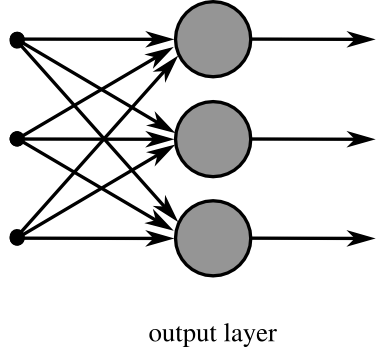源:維基百科神經網路

# 範數

• 範數L1: 公式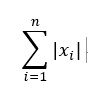，x / x絕對值總和，例如，p1(2, 0)和p2(1, 1)的L1範數，p1範數 = 2，p2範數 = 2，使用L1正規化得到的值不是那麼的穩定，因範數L1的解容易相同，如p1.p2容易凝合若要做反向傳播(反推)時就會受影響，比較適合處理稀疏性問題。

• 範數L2: 公式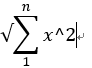，x / x^2總和，例如，p1(2, 0)和p2(1, 1)的L1範數，p1範數 = 2，p2範數 = sqrt(2)，由範例可知L2可以解決L1凝合問題。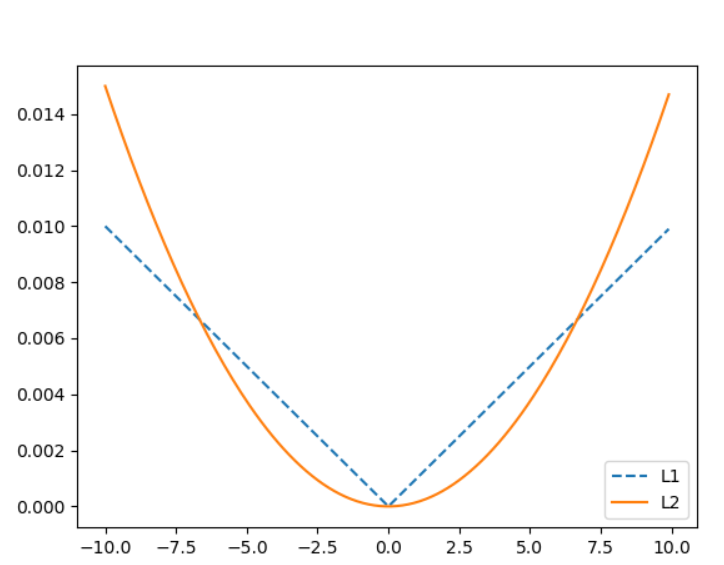# 數學e

### 2的對數表

• 第一項 2^1 = 1
• 第二項 2^2 = 4
• 第三項 2^3 = 8
• 第四項 2^4 = 16
• 第五項 2^5 = 32
當平常我們在計算4 * 8 = 32時候，在這對數表裡就等於第二項 + 第三項 = 第五項(2 + 3 = 5)，也就是我們平常所說的基底一樣的指數的相乘只需要將指數相加。

### e的起源

• 納皮爾想出了一個對數公式為x = b^y = (1 - 1 / 10^8)^y，我們實際計算一次如下圖，它變化非常小幾乎能在這張對數表找到我們目前所需要的"小數精準對數數字"。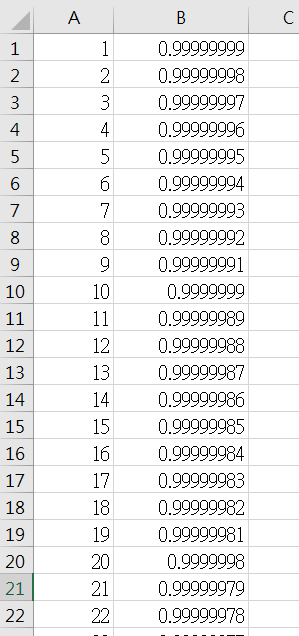• 銀行在使用期數計算利息時也運用到類似的公式，例如，假設我們利率為100%(這裡設定100%，推導公式先忽略)
一年一期：金額 = 本金 * (1 + 1 / 1)
一年二期：金額 = 本金 * (1 + 1 / 2) * (1 + 1 / 2)
一年三期：金額 = 本金 * (1 + 1 / 3) * (1 + 1 / 3) * (1 + 1 / 3)
一年N期：金額 = 本金 * (1 + 1 / N)^N

### 自然對數e

• 尤拉證明了lim n->無限 = (1 + 1 / n)^n = 2.71828182846....命名為e，代表著e是個收斂函數，表示你可以製作出0.00000000000...1的對數表，因為它的小數點精準度和趨近無限的收斂特性(無限分割)，還有它的穩定性，轉換後約差0.06%，可以使數值增大又相對穩定如下圖比較，微分時候也容易許多，我想這就是機器學習和期它工程為何都要使用它的原因，而與loge相對的是ln，公式為e^x = y，x = ln y，也就是log2.71828182846.. = ln(1)。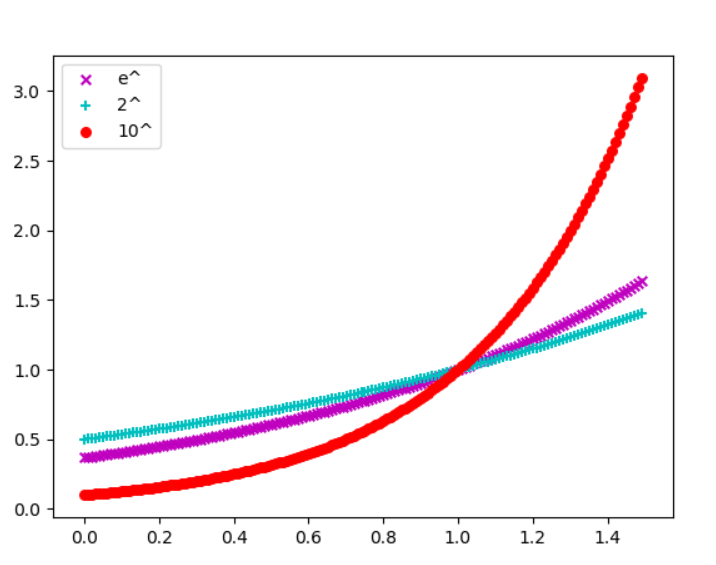上述簡單介紹e，關於e的資訊還有非常多用途可上維基百科查詢。

# 梯度下降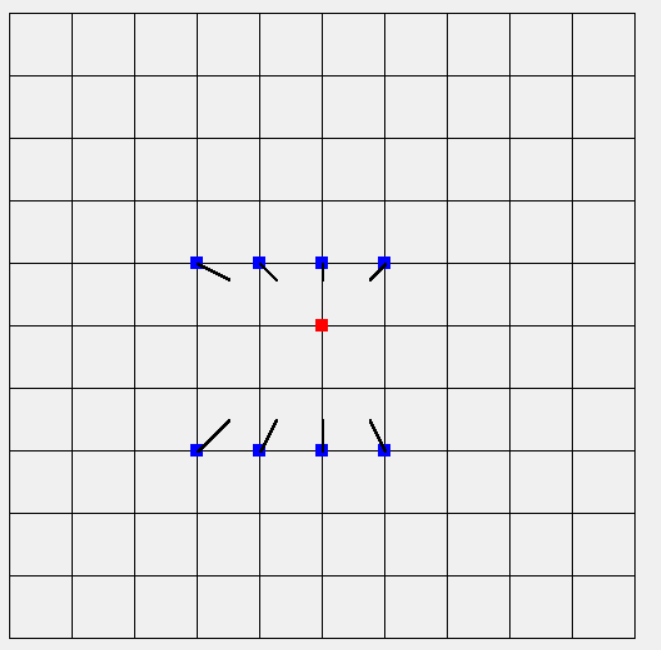• SGD:公式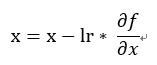，原始的x(權重) - 訓練值 * 取得x在神經網路的變化(偏微分)，但若方向原始不是只向最小值(二維平面解釋:權重差異極大導致箭頭幾乎貼近x軸或y軸)，例如歐萊里書籍提到的f(x, y)1 / 20 * x^2 + y^2 ，我們使用上次用C#來實作，多新增一個Fun2如下方程式碼取代原先的Fun1，結果如圖一，很明顯箭頭方向指向的地方差別非常大，會導致訓練必須花費更多時間甚至無法達到最好結果如圖二(也可說它不平滑)。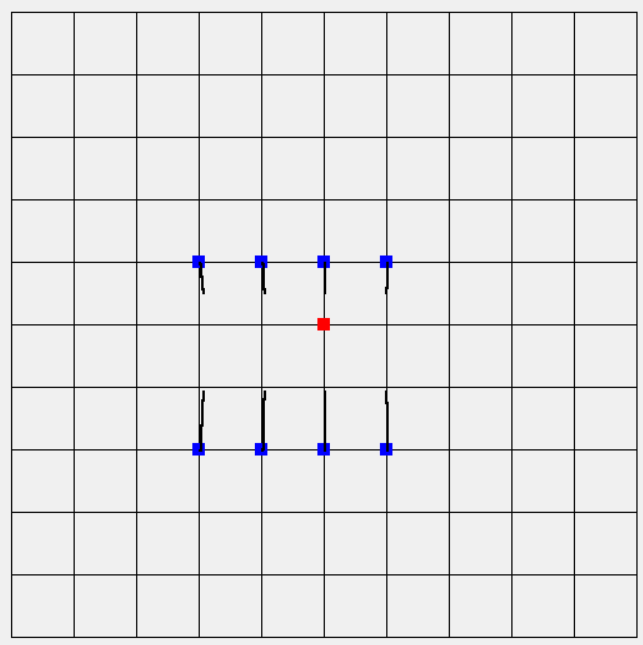圖一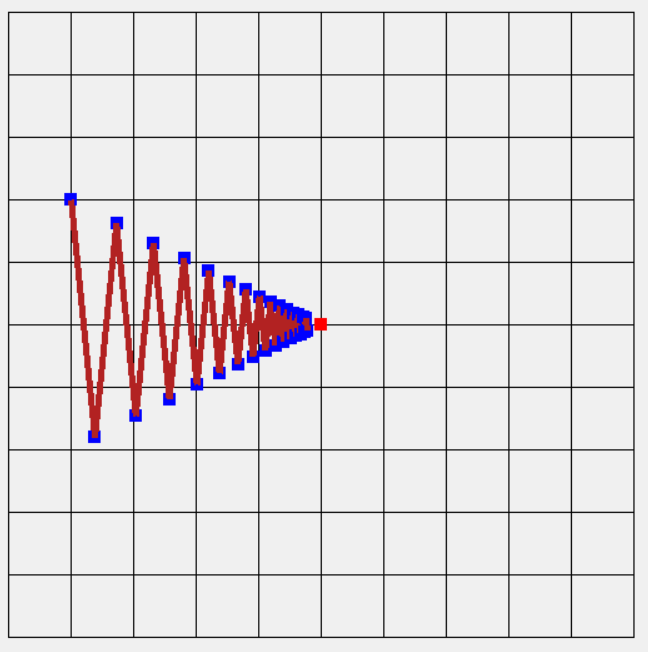``````class Function2 : Function
{
public float Formula(float x, float y)
{
return x * x / 20.0f + y * y;
}

// 手動計算微分，圖二使用
public PointF2D DiffFormula(float x, float y)
{
return new PointF2D(x / 10.0f, 2.0f * y);
}
}

// 圖二使用繪製梯度下降
private void DrawSGD(Graphics graphics, DrawF draw)
{
ArrayList history = new ArrayList();
PointF2D point = new PointF2D(-4.0f, 2.0f);
Function2 fun = new Function2();
SGD optimizer = new SGD(0.95f);

for (int index = 0; index < 30; index++)
{
PointF2D xyPoint = draw.getBlockPoint(point.X, point.Y);
PointF2D diff = fun.DiffFormula(point.X, point.Y);
optimizer.Update(point, diff);
}

PointF2D prePoint = ((PointF2D)history);

for (int index = 0; index < 30; index++)
{
draw.drawPoint(graphics, Brushes.Blue, ((PointF2D)history[index]));
draw.drawLine(graphics, prePoint, ((PointF2D)history[index]));
prePoint = ((PointF2D)history[index]);
}
}

// 圖二使用繪製梯度下降法函數
class SGD
{
private float _lr;

public SGD(float lr)
{
_lr = lr;
}

public void Update(PointF2D point, PointF2D grad)
{
point.X = point.X - grad.X * _lr;
point.Y = point.Y - grad.Y * _lr;
}
}
``````
• Momentum:公式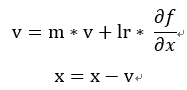，這裡主要加入了物理的摩擦力想法，多了一個m參數主要用來控制上一個動作(梯度)的摩擦力，就像你把一個東西往前推兩次，第一次向前推物體移動，若直接推第二次物體他還會加上原先的損失受力(已經在移動原先受力已經損耗)，利用這原理可讓訓練更快也讓函數更加平滑，這裡可以看出平滑函數的浮動和SGD相比差異。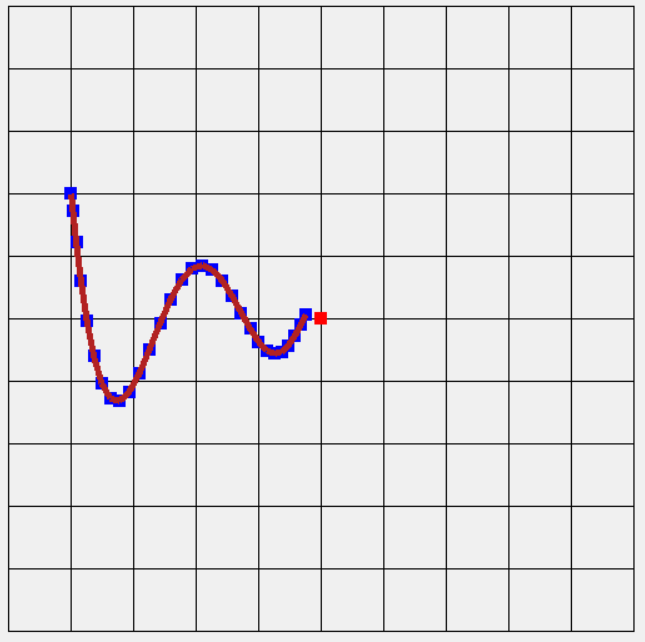``````// 原本SGD只需換成Momentum
private void DrawMomentum(Graphics graphics, DrawF draw)
{
ArrayList history = new ArrayList();
PointF2D point = new PointF2D(-4.0f, 2.0f);
Function2 fun = new Function2();
Momentum optimizer = new Momentum(0.07f, 0.9f);

for (int index = 0; index < 30; index++)
{
PointF2D xyPoint = draw.getBlockPoint(point.X, point.Y);
PointF2D diff = fun.DiffFormula(point.X, point.Y);
optimizer.Update(point, diff);
}

PointF2D prePoint = ((PointF2D)history);

for (int index = 0; index < 30; index++)
{
draw.drawPoint(graphics, Brushes.Blue, ((PointF2D)history[index]));
draw.drawLine(graphics, prePoint, ((PointF2D)history[index]));
prePoint = ((PointF2D)history[index]);
}
}

// Momentum函數
class Momentum
{
private float _lr;
private float _m;
private float[] _v;

public Momentum(float lr, float m)
{
_lr = lr;
_m = m;
_v = new float;
}

public void Update(PointF2D point, PointF2D grad)
{
_v = _m * _v + grad.X * _lr;
_v = _m * _v + grad.Y * _lr;
point.X = point.X - _v;
point.Y = point.Y - _v;
}
}
``````
• AdaGrad: 公式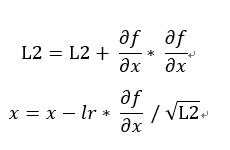，這時候就可以使用L2範化，還記得上面L2範化圖嗎，它就是利用此方法所畫出的圖形，更新到最後會趨近於0(等於0)，這方法可讓學習依L2相對的"比例"衰減，與過去梯度的平方來取得相對應的相對的比例，可看到下圖更新速度和平滑度都是不錯的。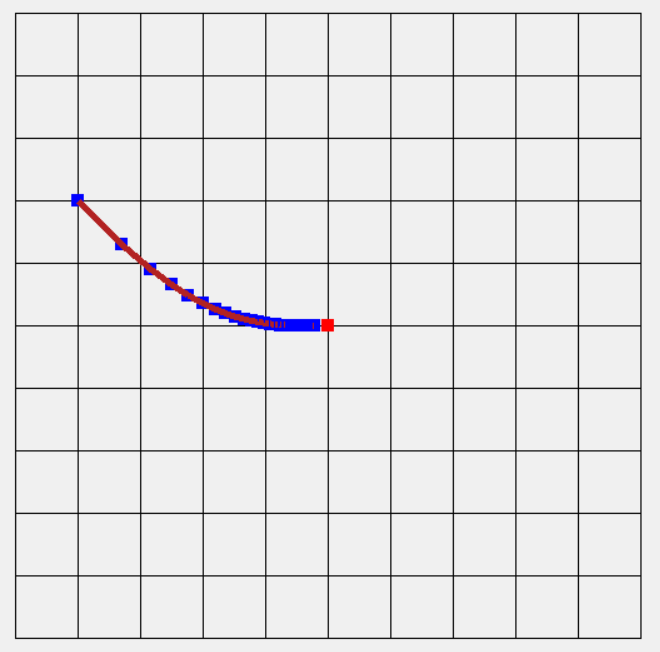``````private void DrawAdaGrad(Graphics graphics, DrawF draw)
{
ArrayList history = new ArrayList();
PointF2D point = new PointF2D(-4.0f, 2.0f);
Function2 fun = new Function2();

for (int index = 0; index < 30; index++)
{
PointF2D xyPoint = draw.getBlockPoint(point.X, point.Y);
PointF2D diff = fun.DiffFormula(point.X, point.Y);
optimizer.Update(point, diff);
}

PointF2D prePoint = ((PointF2D)history);

for (int index = 0; index < 30; index++)
{
draw.drawPoint(graphics, Brushes.Blue, ((PointF2D)history[index]));
draw.drawLine(graphics, prePoint, ((PointF2D)history[index]));
prePoint = ((PointF2D)history[index]);
}
}

{
private float _lr;
private float[] _l2;

{
_lr = lr;
_l2 = new float;
}

public void Update(PointF2D point, PointF2D grad)
{
point.X = point.X - _lr * grad.X / (float)Math.Sqrt(_l2 + 0.0000001f);
point.Y = point.Y - _lr * grad.Y / (float)Math.Sqrt(_l2 + 0.0000001f);
}
}
``````
• RMSProp: 公式，AdaGrad的改進，這裡多了一個q衰減率，每次學習都會讓過去的權重依一定比例衰減，然而就比原先AdaGrad的函數更加多變，更適合拿來處理多變函數的學習(與原先函數相比)，如下圖衰減率0.9(每次損失倍率0.1)，學習率比原先的AdaGrad還要小，點和點的間距也明顯縮小，可以讓學習更加穩定。這裡衰減率的想法就像聲波光波"傳遞"時會衰減，依人工智慧角度來看就是記憶力，會隨著時間消逝而慢慢遺忘。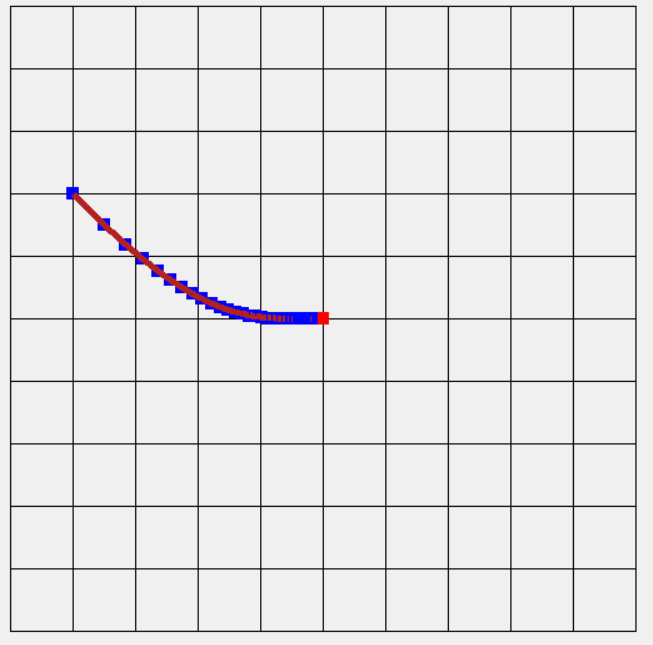``````private void DrawRMSProp(Graphics graphics, DrawF draw)
{
ArrayList history = new ArrayList();
PointF2D point = new PointF2D(-4.0f, 2.0f);
Function2 fun = new Function2();
RMSProp optimizer = new RMSProp(0.5f, 0.9f);

for (int index = 0; index < 30; index++)
{
PointF2D xyPoint = draw.getBlockPoint(point.X, point.Y);
PointF2D diff = fun.DiffFormula(point.X, point.Y);
optimizer.Update(point, diff);
}

PointF2D prePoint = ((PointF2D)history);

for (int index = 0; index < 30; index++)
{
draw.drawPoint(graphics, Brushes.Blue, ((PointF2D)history[index]));
draw.drawLine(graphics, prePoint, ((PointF2D)history[index]));
prePoint = ((PointF2D)history[index]);
}
}

class RMSProp
{
private float _lr;
private float _q;
private float[] _sum;

public RMSProp(float lr, float q)
{
_lr = lr;
_q = q;
_sum = new float;
}

public void Update(PointF2D point, PointF2D grad)
{
point.X = point.X - _lr * grad.X / (float)Math.Sqrt(_sum);
point.Y = point.Y - _lr * grad.Y / (float)Math.Sqrt(_sum);
}
}
``````
• Adam: 公式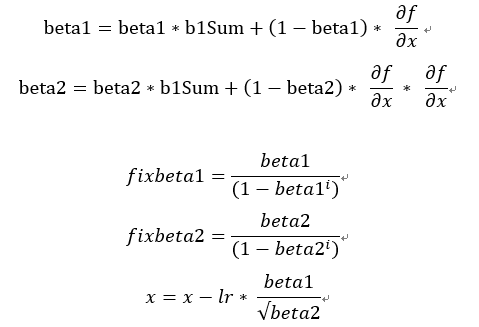，Adam將RMSProp和Momentum結合並計算偏差值，這裡運用到統計學計算方式未來有機會學習到在介紹真正原理。先來講解一下自己本身看法，beta1主要控制目前梯度加總的衰減率，beta2主要控制L2範數衰減率。然而這裡我們要計算偏差值，我們希望在N步前的比例是beta，近N步比例是(1 - beta)，然而需要計算近N步所占的比率，當你疊代1次我們所占的比例會乘上beta，因此偏差得到beta / (1 - beta^疊代次數)，最後x減掉學習率乘上L2正規化lr * fixbeta1 / sqrt(fixbeta2)。簡單來說我們主要利用過去梯度，在計算從一開始到現在的疊代的比例，正規化後來去穩定更新梯度。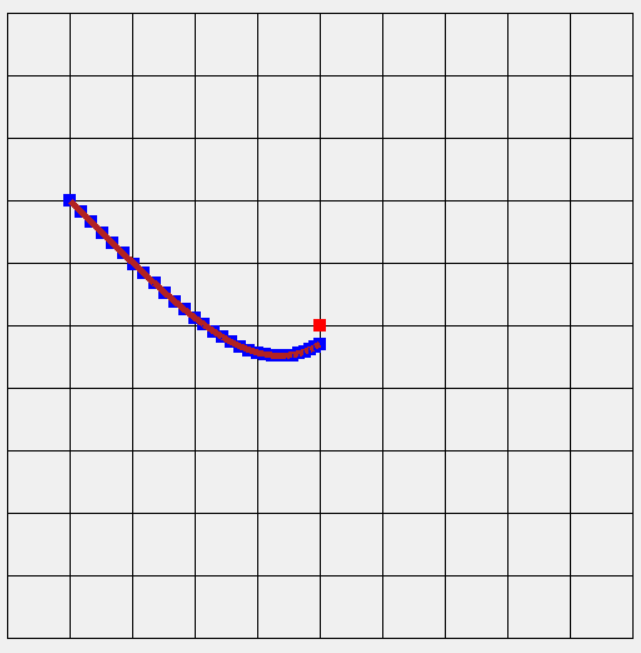``````private void DrawAdam(Graphics graphics, DrawF draw)
{
ArrayList history = new ArrayList();
PointF2D point = new PointF2D(-4.0f, 2.0f);
Function2 fun = new Function2();

for (int index = 0; index < 30; index++)
{
PointF2D xyPoint = draw.getBlockPoint(point.X, point.Y);
PointF2D diff = fun.DiffFormula(point.X, point.Y);
optimizer.Update(point, diff);
}

PointF2D prePoint = ((PointF2D)history);

for (int index = 0; index < 30; index++)
{
draw.drawPoint(graphics, Brushes.Blue, ((PointF2D)history[index]));
draw.drawLine(graphics, prePoint, ((PointF2D)history[index]));
prePoint = ((PointF2D)history[index]);
}
}

{
private float _lr;
private float _beta1;
private float _beta2;
private float[] _b1Sum;
private float[] _b2Sum;
private int _iter;
public Adam(float lr, float beta1, float beta2)
{
_lr = lr;
_beta1 = beta1;
_beta2 = beta2;
_b1Sum = new float;
_b2Sum = new float;
_iter = 1;
}

public void Update(PointF2D point, PointF2D grad)
{
_b1Sum = _beta1 * _b1Sum + (1.0f - _beta1) * grad.X;
_b1Sum = _beta1 * _b1Sum + (1.0f - _beta1) * grad.Y;

_b2Sum = _beta2 * _b2Sum + (1.0f - _beta2) * grad.X * grad.X;
_b2Sum = _beta2 * _b2Sum + (1.0f - _beta2) * grad.Y * grad.Y;

float[] b1Fix = new float;
b1Fix = _b1Sum / (1.0f - (float)Math.Pow(_beta1, _iter) + 0.00000001f);
b1Fix = _b1Sum / (1.0f - (float)Math.Pow(_beta1, _iter) + 0.00000001f);

float[] b2Fix = new float;
b2Fix = _b2Sum / (1.0f - (float)Math.Pow(_beta2, _iter) + 0.00000001f);
b2Fix = _b2Sum / (1.0f - (float)Math.Pow(_beta2, _iter) + 0.00000001f);

point.X = point.X - _lr * b1Fix / ((float)Math.Sqrt(b2Fix) + 0.00000001f);
point.Y = point.Y - _lr * b1Fix / ((float)Math.Sqrt(b2Fix) + 0.00000001f);
_iter++;
}
}
``````

# 活化函數

• Sigmoid函數:公式為1 / (1 + exp(-x))，主要分布於0 ~ 1但到某個值(好像是4.X和-4.X)會非常趨近1和0。這時候超過某個值都會是相同的，也就是我們會失去一些原先的訊息。

• Relu函數:公式為x < 0輸出0，x > 0輸出x，主要分布0~N，把小於0的值都設定為0，大於直接輸出。但這時候我們將會失去負號的相關資訊，然而就有人提出進階的Relu想法(合併Sigmoid函數等等)。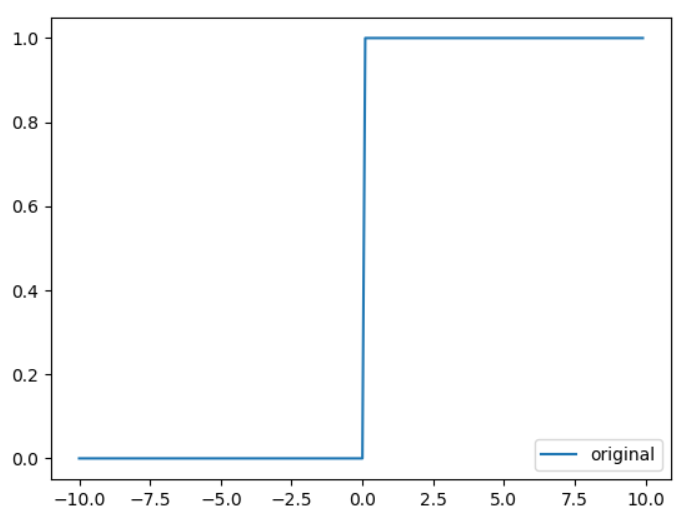階梯函數Sigmoid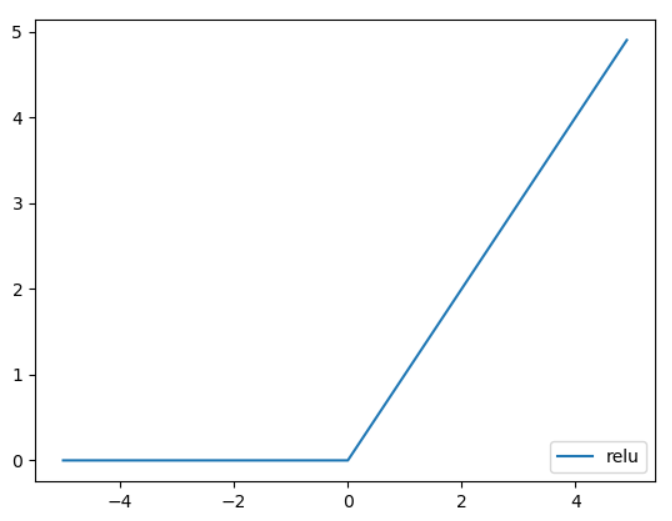Relu

# 輸出層活化函數

• 回歸問題我們使用恆等函數(直接輸出)。
• 分類問題我們使用softmax函數，公式f(x, i) = exp(xi) / sigma 1~n(exp(x))，i為第i個x，n為x總數量，主要使用指數歸一化，softmax比例差距會放大使學習更快等等，由Sigmoid平滑函數延伸而來，利用平滑特性給予相對的權重，將目前機率放大，e對小數指數也有比較好的對照表，也可以使用其它的但我結果可能不一定會更好。

# 損失函數

## 損失函數

### 均方誤差函數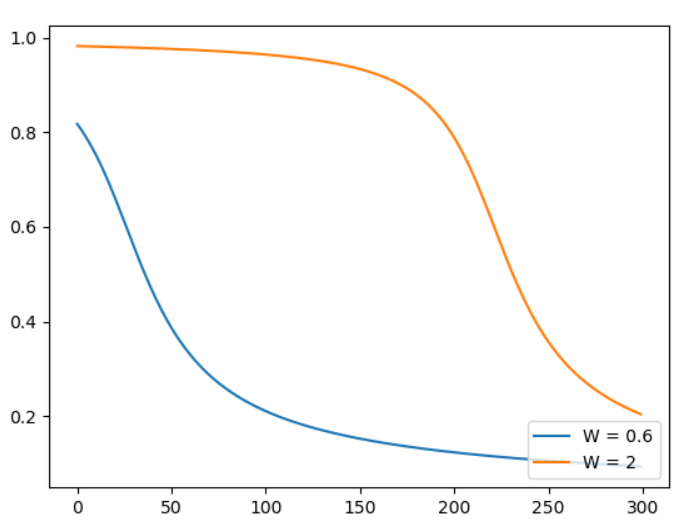``````import numpy as np
import matplotlib.pylab as plt

def sigmoid(x):
return 1 / (1 + np.exp(-x))

def fun(x, w, b):
return x * w + b

def loss(x, w, b):
a = fun(x, w, b)
y = sigmoid(a)
return 0.5 * (y ** 2)

h = 1e-2

temp = w
w = temp + h
fun1 = loss(x, w, b)
w = temp - h
fun2 = loss(x, w, b)
w = temp
grad['dW'] = (fun1 - fun2) / (2 * h)

temp = b
b = temp + h
fun1 = loss(x, w, b)
b = temp - h
fun2 = loss(x, w, b)
b = temp
grad['db'] = (fun1 - fun2) / (2 * h)

def tran(x, w, b):
lr = 0.15
times = 300

history_y = []

for index in range(300):
a = fun(x, w, b)
y = sigmoid(a)
history_y.append(y)
return history_y

if __name__ == '__main__':
y1 = tran(1, 0.6, 0.9)
y2 = tran(1, 2, 2)
plt.plot(np.arange(0, 300, 1), np.array(y1), label = 'W = 0.6')
plt.plot(np.arange(0, 300, 1), np.array(y2), label = 'W = 2')
plt.legend()
plt.show()
``````

### 交叉熵函數

• 黃:第一層->左(拿1次)->第二層->左(拿2次)->黃色 2次
• 綠:第一層->左(拿1次)->第二層->右(拿2次)->綠色 2次
• 紅:第一層->右(拿1次)->第二層->左(拿2次)->紅色 2次
• 藍:第一層->右(拿1次)->第二層->右(拿2次)->藍色 2次
訊息熵 = 1 / 4 * 2 + 1 / 4 * 2 + 1 / 4 * 2 + 1 / 4 * 2 = 2

• 黃:第一層->左(拿1次)->黃色 1次
• 綠:第一層->右(拿1次)->第二層->左(拿2次)->綠色 2次
• 紅:第一層->右(拿1次)->第二層->右(拿2次)->第三層->左(拿3次)->紅色 3次
• 藍:第一層->右(拿1次)->第二層->右(拿2次)->第三層->右(拿3次)->藍色 3次
訊息熵 = 1 / 2 * 1 + 1 / 4 * 2 + 1 / 8 * 3 + 1 / 8 * 3 = 1.75
因此推導出此公式信息熵 = sigma 機率 * log2(1 / 機率)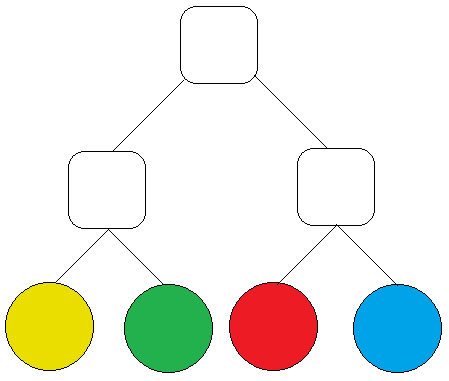圖1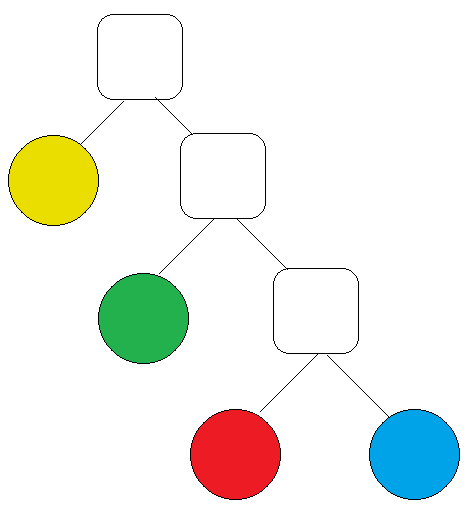圖2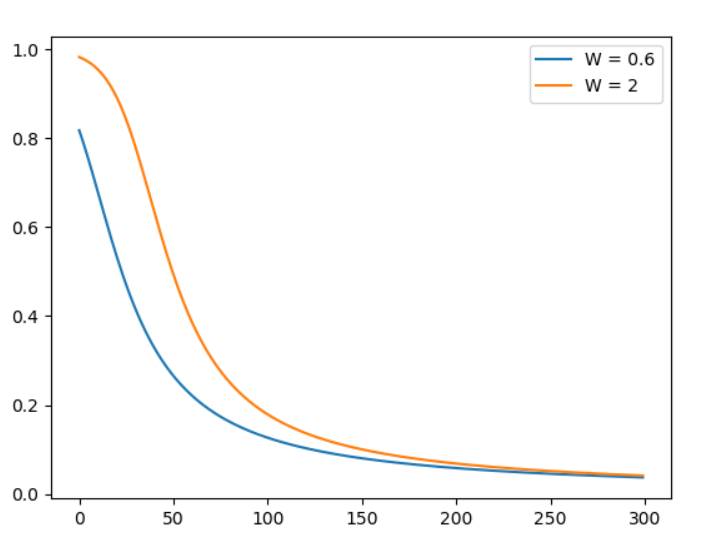``````# 取代平均方差，這裡我們測試，只有一個答案，所以用原來的交叉熵公式
def loss(x, w, b):
a = fun(x, w, b)
y = sigmoid(a)
return -np.sum(0 * np.log(y) + (1 - 0) * np.log((1 - y) + 1e-7))
``````

# 參考網址

### 1 則留言

0Kevin iT邦新手 4 級 ‧ 2018-08-12 10:07:14 檢舉

fysh711426 iT邦研究生 2 級 ‧ 2018-08-14 00:24:37 檢舉Kevin iT邦新手 4 級 ‧ 2018-08-14 18:43:35 檢舉